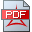The high level OVERVIEW page content to the topics RH, NSE, YME, GUT until end of 2017 are summaried inRH, YME, NSE overview page, 2012-2017

A. An alternative entire Zeta function representation and its related duality equation

The definition of the alternative entire Zeta function is built on the Hilbert transformed Gauss-Weierstrass function to take advantage of one of its properties, that each Hilbert transformed function has vanishing constant Fourier term.

The alternative entire Zeta function representation enables an alternative Riemann power series expansion as described in (EdH) 1.8. The Riemann series expansion is the baseline to define the Li criterion and power series representation of related functions. As tan(...)/(...) has no zeros in the critical stripe, the power series representation of the alternatively proposed entire Zeta function provides a new baseline to verify the Li criterion.

The Li criterion is related to the Berry-Keating conjecture (the physical interpretation of the Hilbert-Polya conjecture), which is about eigenvalues of self adjoint operators. In this context we emphasis a second property of the Hilbert transformation operator H, i.e. the commutator operator (xH-Hx)(g) vanishes, in case the function or distribution g has a vanishing constant Fourier term. We mention in this context, that the wave function of the ground state of the harmonic quantum oscillator is given by the Gauss-Weierstrass function, which builds the other side of the (unfortunate) "coin", that the quantum mechanics ladder operators are non hermitean in the standard L(2) domain framework. In the ground state energy section we propose a less regular Hilbert space domain H(-b) of alternative ladder operators and corresponding wave functions of their corresponding ground states (also in the sense of the above) to take advantage of the two Hilbert transformation properties above. The extended domain enables  the definition of modified ladder operators in a way, that they now can incorporate a continuous spectrum on the complementary closed subspace domain (H(-b)-H(0), whereby the projection onto the standard L(2) Hilbert space generates the above two L(2)-wave functions of their ground state.

The central new technical element is a change in the underlying Hilbert space framework, i.e. the standard L(2)-Hilbert space framework is replaced by the distributional Hilbert space H(-1/2). This has two immediate consequences

- an alternative Schrödinger momentum operator can be defined, whereby the complementary closed space H(-1/2)-H(0) enables an alternative way to model "wave functions" of the ground state energy resp. condensates, superfluids & superconductivity (J. F. Annett)

- an alternative Dirac function model can be defined, whereby the regularity of the "defining" H(-1/2) Hilbert space is (now) independent from the space dimension "n" and, at the same time, more regular than the Dirac distribution "function" itself even for the space dimension n=1 (!!). In other words, an alternative modelling framework for current "physical" applications of the Dirac "function" is provided with (slightly, but essentially) reduced mathematical model required regularity assumptions to current state of the art theory, which is now (newly) independent from the mathematical model`s space-time dimension.

The new framework is proposed to be applied for the other two Millenium problems in scope of this Homepage, i.e. the NSE Serrin gap (note that the "pressure" and the "energy density" do have same unit of measure) and the YME mass gap problems.

B. The alternative Ei(x)-function, Farey series and the Goldbach conjectures

Two central concepts in the proposed Kummer function based Zeta function theory are

- an appropriate distributional framework for Ramanujan's generalized distributional Fourier series representation of cot(x) with its relationship to the -ln(2sin(x))-function (e.g. lemmata 2.4, 3.3, lemma H4, supporting lemmata 44-47, opportunity lemmata O5-7, O22, O27, O37, note O7)

- an alternative Ei(x) function (and its corresponding alternative li(x)-function) defined by the special Kummer function F(1/2,3/2,x) (e.g. lemmata K2, CF1, S7) with identical convergence behavior as Ei(x), e.g. remark 2.3

The zeros of F(1/2,3/2,z) (whereby Re(z)>1/2, note O5, lemma A4) enable the definition of a corresponding Hilbert scale framework in line with the domain of the generalized distributional Fourier series representation of the cot(x)-function. This framework is proposed to be applied to answer both Goldbach conjectures positively, whereby the modified Farey series (not building on Weyl sums) builds a central concept; we note that Vinogradov's  solution concept provides the required estimates for the major arcs, while the corresponding minor arcs estimates (based on Weyl sums) are not sufficient to prove both Goldbach conjectures; the fundamental issue for the minor arcs estimates is the fact that there is no information taken into account from the Goldbach problem itself; Vinograd's minor arcs estimates are purely based on Weyl sums properties, i.e they are valid independently from the to be solved problem and, at the same time, jeopardizing Vinograd's solution idea.

Regarding the above the following references (Nachrichten der Gesellschaft der Wissenschaften zu Göttingen) are appended:

C. Transcendental numbers and Kummer functions

Literature

D. A Whittaker function based characterization of the alternative Ei(x) function

With respect to the supporting lemma S15 (note O1) we note that corresponding Whittaker functions (and their corresponding relationships to the erf(x)-function) provide a characterization of the alternative Ei(x)-function in the form

M(z) * N(z) = x * F(1/2,3/2,z)

whereby M(z) resp. N(z) denote the Whittaker functions with the parameters (l,m)=(1/4,1/4) resp. (l,m)=(-1/4,-1/4).

E. Music melodies and Ramanujan's generalized distributional Fourier series domain

Music and mathematics might be seen as two different sides of the same coin; and "yes", there is such a coin which is about the music melodies; those lie exactly between the red (Brownian motion) and the white (derivative of the Brownian motion) noise resp. its motion domain lies exactly between the two integer scaled Hilbert space domains of the red and the white noise: